## 9-6 嚙踝蕭嚙踝蕭嚙踝蕭嚙踝蕭嚙踝蕭嚙踝蕭嚙踝蕭嚙踝蕭嚙踝蕭嚙踝蕭嚙踝蕭嚙踝蕭嚙踝蕭嚙踝蕭嚙踝蕭

MATLAB 亦提供了一系列指令，可用來解決三角化（Triangulation）、鄰近點（Nearest Neighbors），及其它計算幾何（Computational Geometry）方面的問題，首先我們解釋何謂 Delaunay 三角化（Trangulation）。給定一組 X-Y 平面上的點，Delaunay 指令可傳回一組由這些點所形成的三角形，而且任一點均不會位於任一個三角形的外接圓之內。為展示 Delaunay 的用法，首先我們讀入一組 X-Y平面上的資料點，並以散佈圖的方式來表示：

Example 1: 09-內插法/seamount01.mload seamount.mat plot(x, y, '.');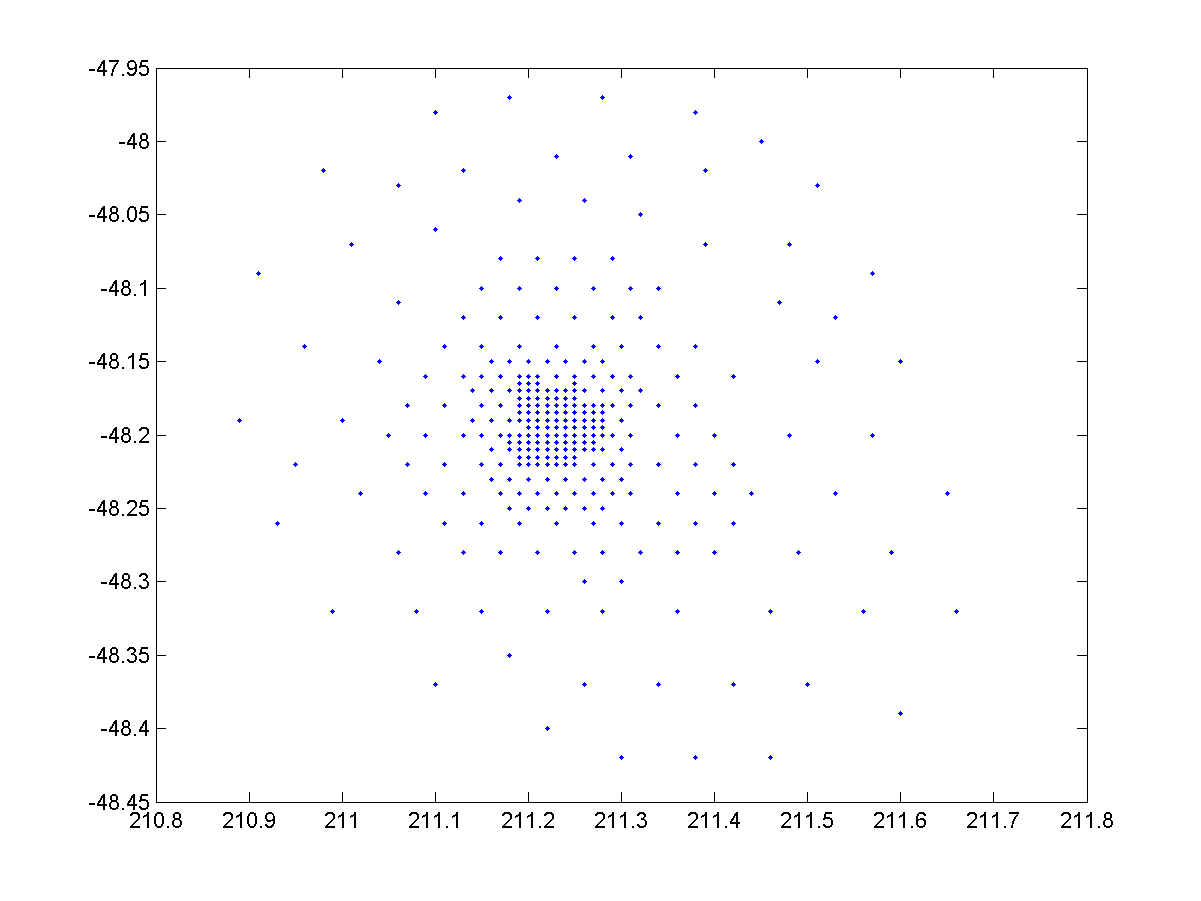Example 2: 09-內插法/triplot01.mload seamount.mat tri = delaunay(x, y); triplot(tri, x, y); hold on, plot(x, y, '.r'); hold off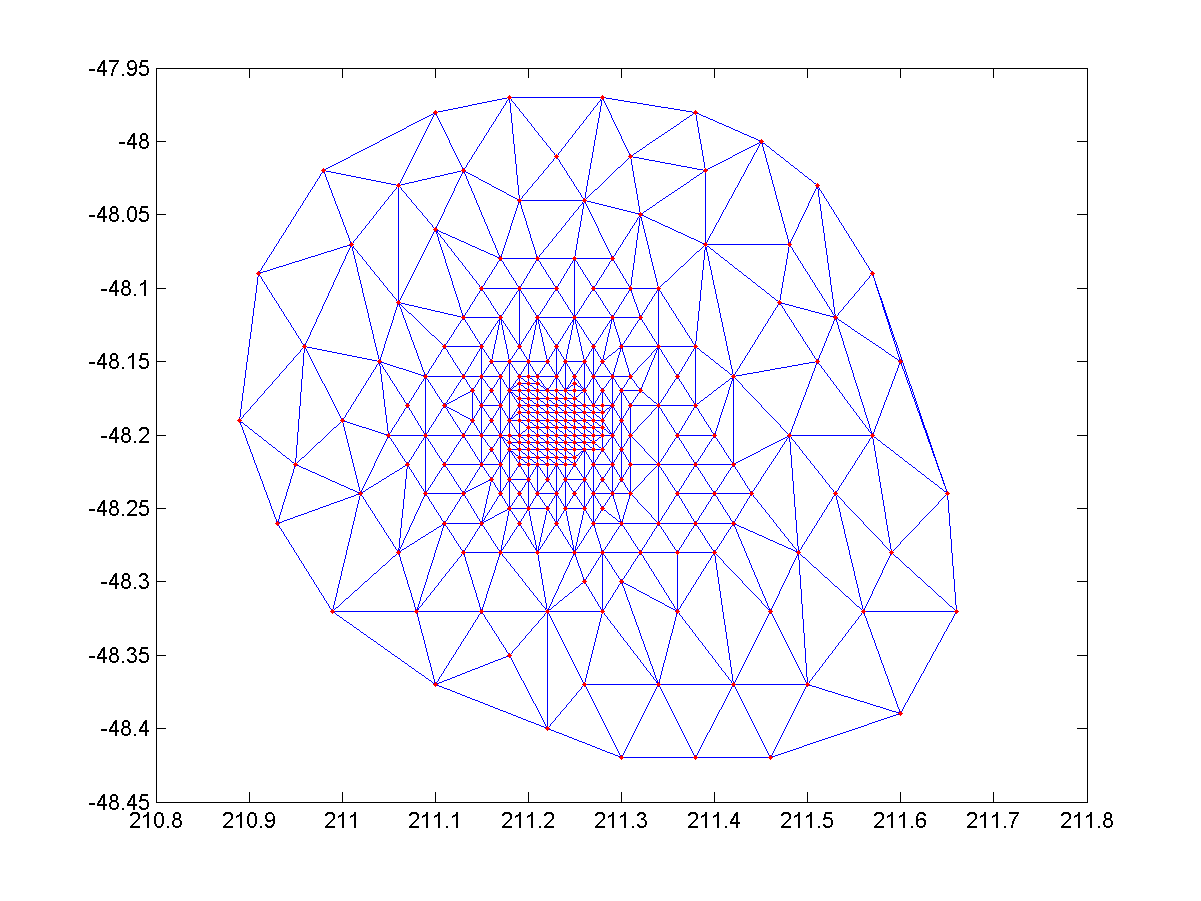Example 3: 09-內插法/trisurf01.mload seamount.mat tri = delaunay(x, y); trisurf(tri, x, y, z); axis tight; colorbar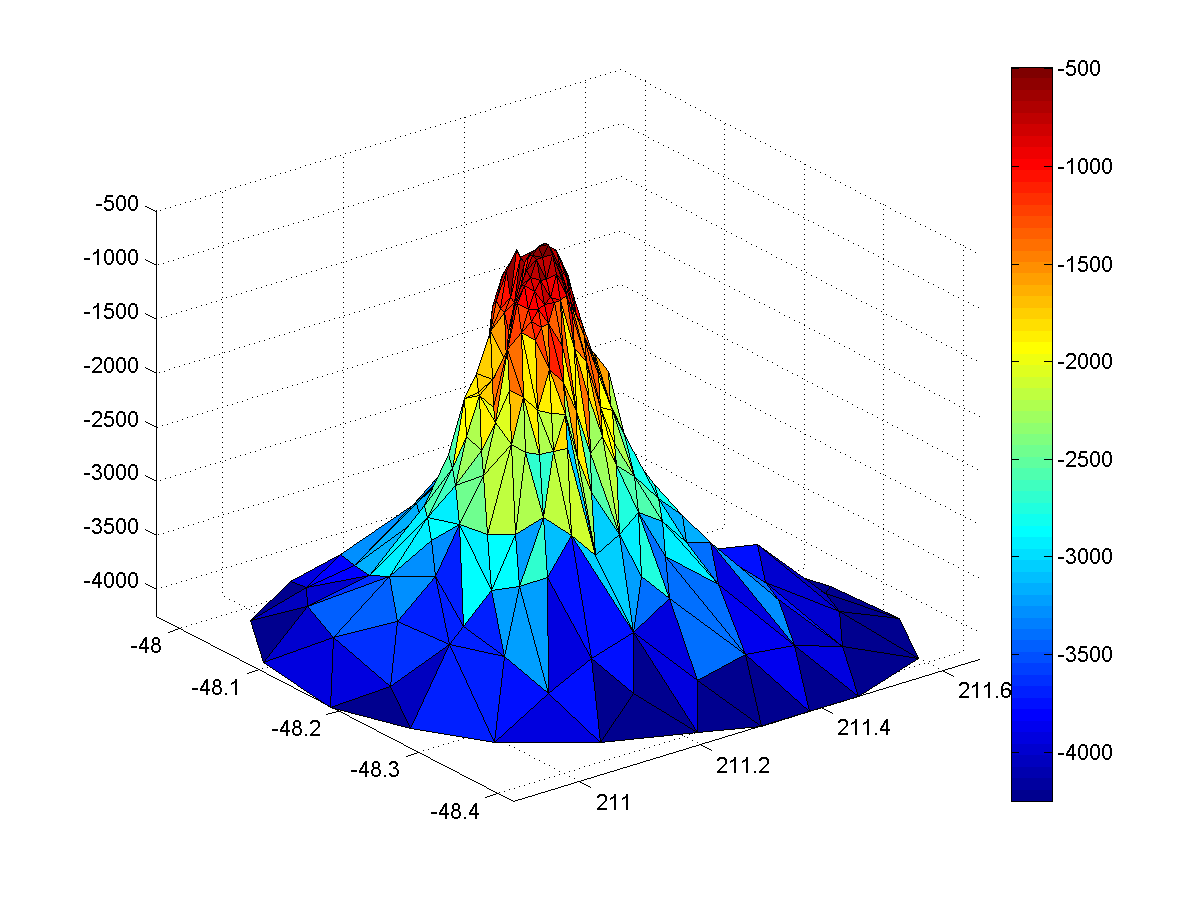Example 4: 09-內插法/tricontour01.mload seamount.mat xi = linspace(min(x), max(x), 50); yi = linspace(min(y), max(y), 50); [xi, yi] = meshgrid(xi, yi); zi = griddata (x, y, z, xi, yi, 'cubic'); [c, h] = contourf(xi, yi, zi, 'b-'); clabel (c, h); colorbar; % 顯示顏色與函數值的對照表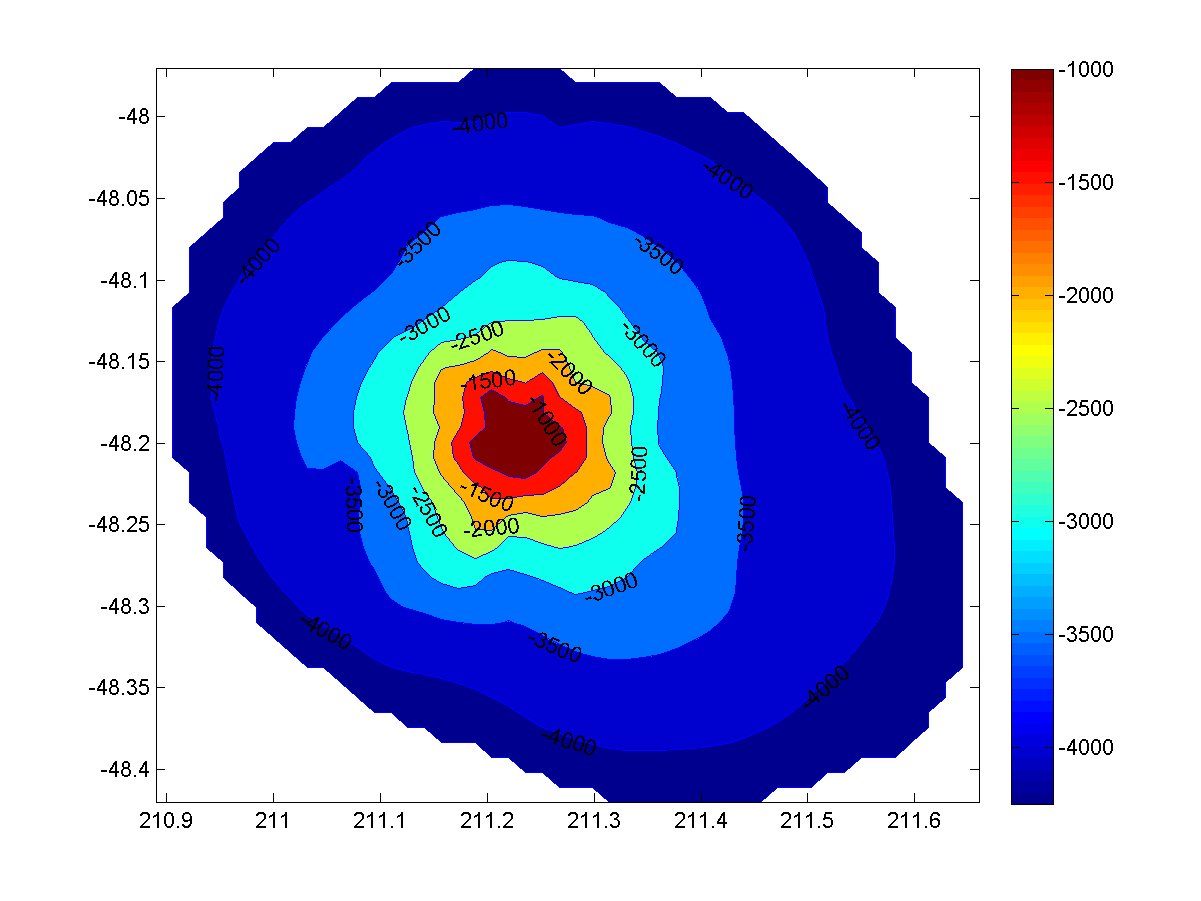• dsearch 指令可找出一給定點的最鄰近資料點
• tsearch 指令可找出一給定點所在之三角形

Voronoi 圖形和 Delaunay 三角化關係非常密切，Voronoi 圖形可將資料點所在的平面畫分成數個多邊形，每一個多邊形只包含一個資料點，而且給定任一點時，此點和其最鄰近的資料點會落於同一個多邊形內。事實上，只要將 Delaunary 所得的各個三角形中，對每一邊畫出垂直平方線，即可得到 Voronoi 圖形。MATLAB 的 voronoi 指令可用來畫出 Voronoi 圖形，舉例如下：

Example 5: 09-內插法/voronoi01.mload seamount.mat voronoi(x, y);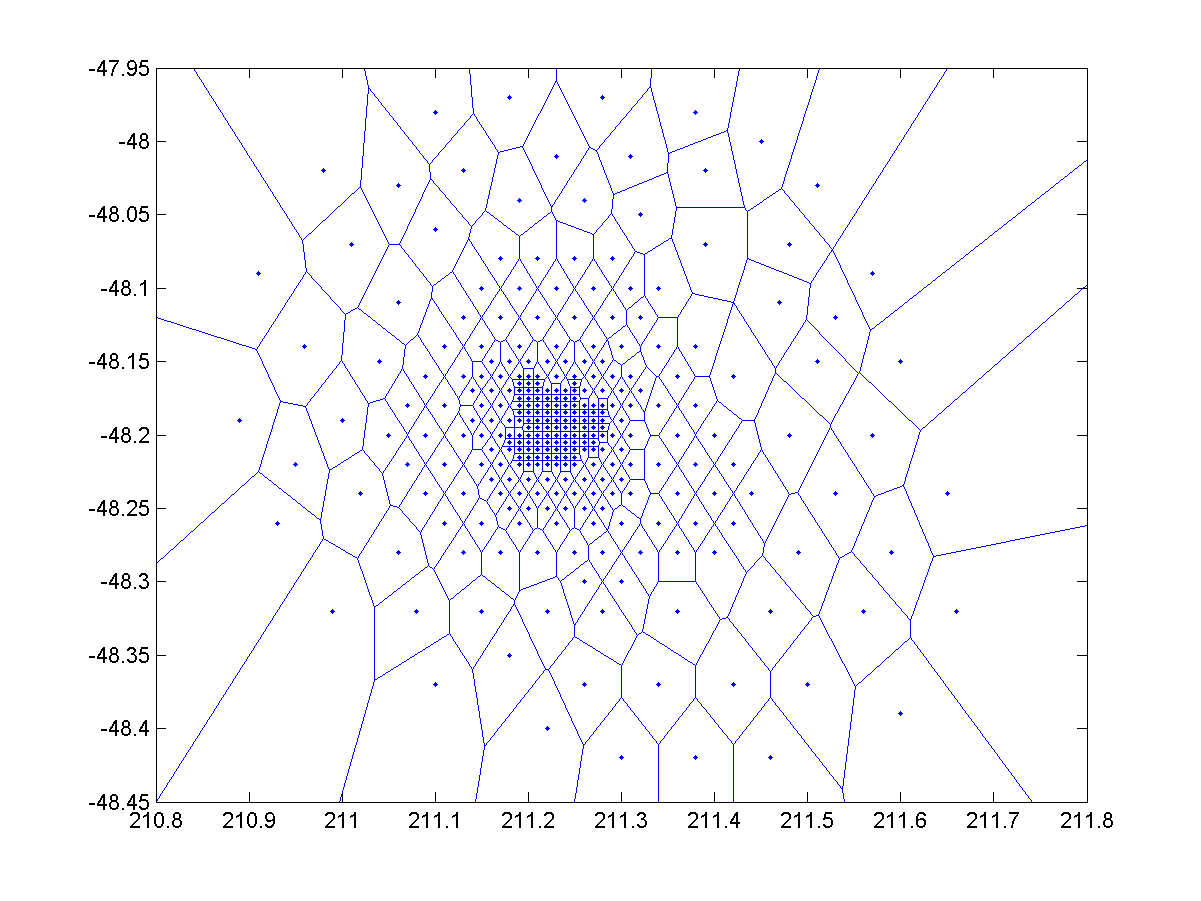Example 6: 09-內插法/voronoi02.mx = rand(20,1); y = rand(20,1); voronoi(x, y, 'b:'); tri = delaunay(x, y); hold on h = trimesh(tri, x, y, 0*x); hold off set(h, 'facecolor', 'none'); axis equal square axis([0 1 0 1]);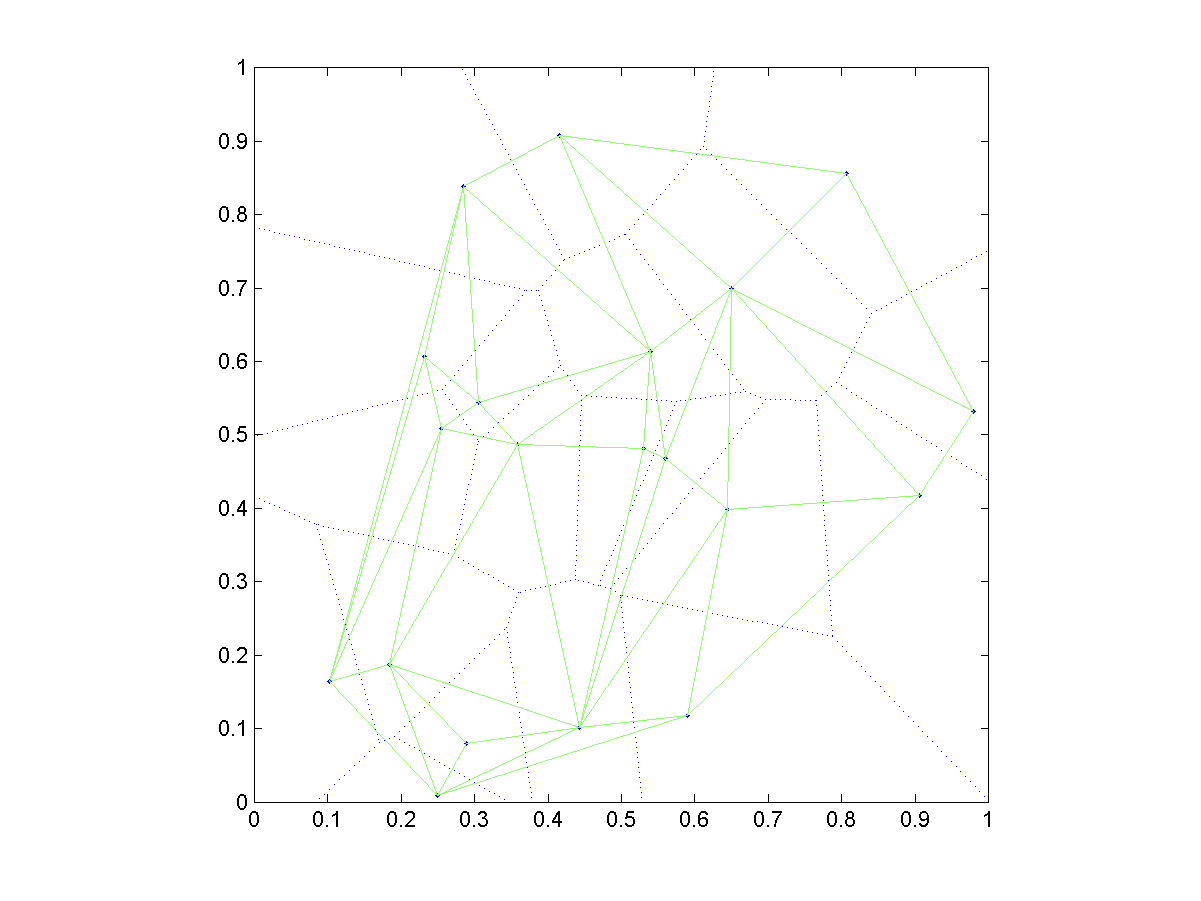Hint

Example 7: 09-內插法/convhull01.mload seamount.mat plot(x, y, '.'); k = convhull(x, y); hold on, plot(x(k), y(k), 'r'), hold off % 畫出最小凸多邊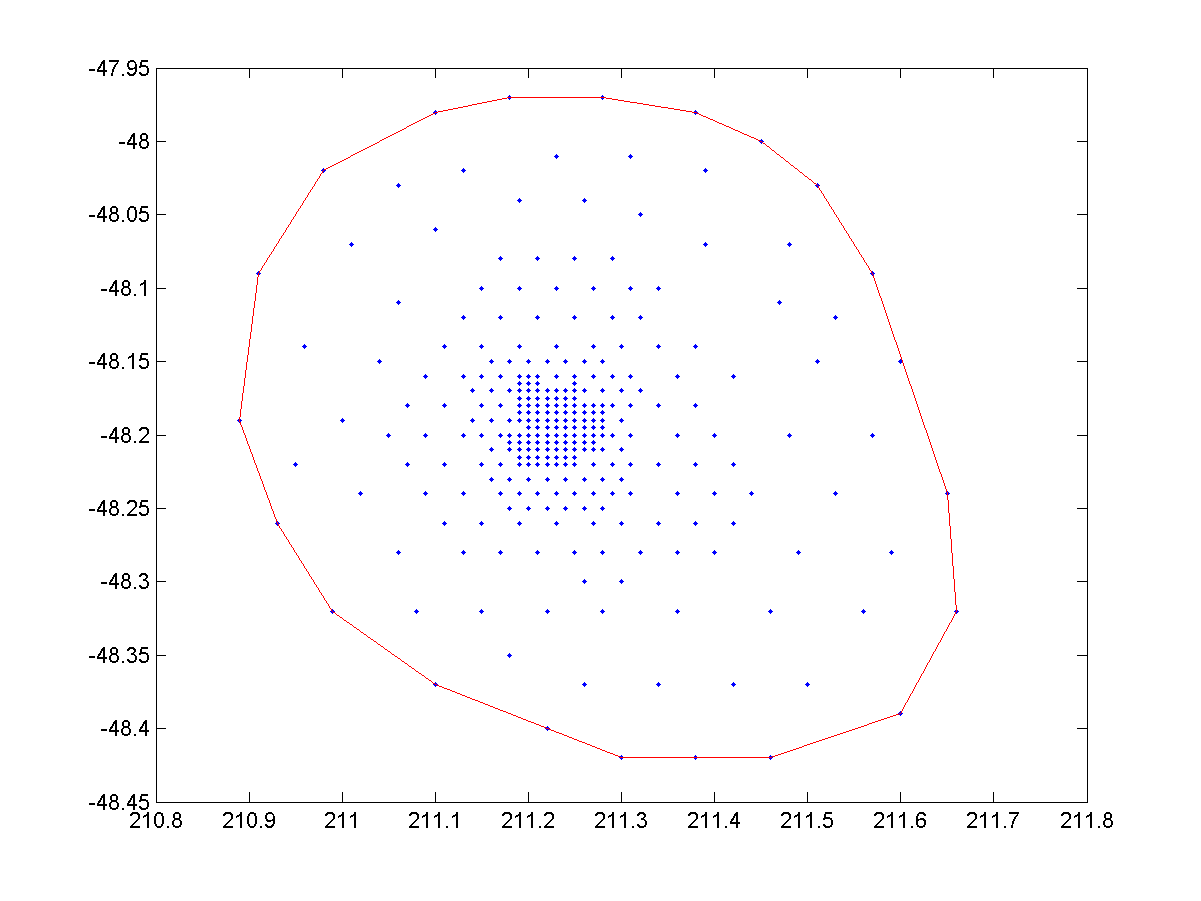Example 8: 09-內插法/inpolygon01.mtheta = linspace(0, 2*pi, 7); xv = cos(theta); yv = sin(theta); x = randn(250,1); y = randn(250,1); in = inpolygon(x, y, xv, yv); plot(xv, yv, 'b', x(in), y(in), 'g.', x(~in), y(~in),'k.'); axis image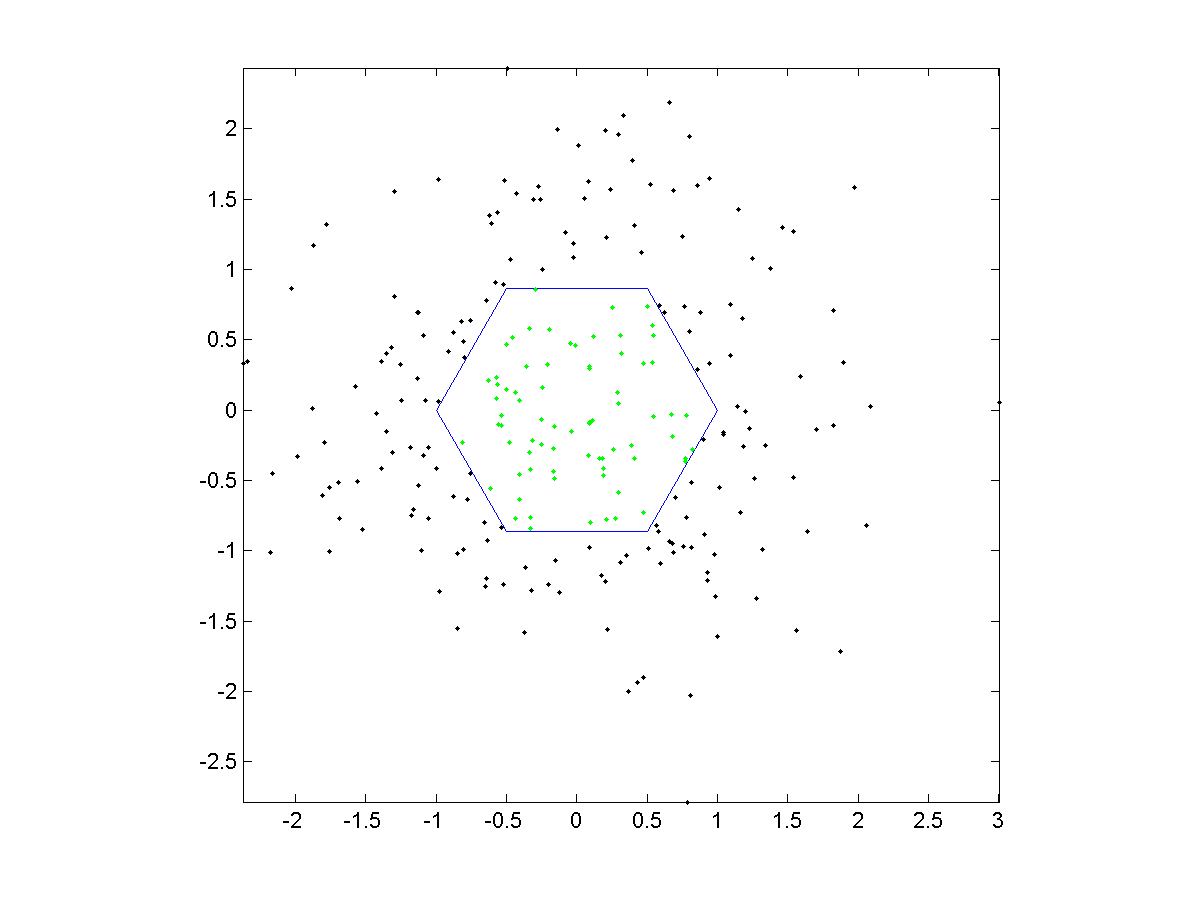MATLAB程式設計：進階篇